• 一.Java的基本資料型別介紹
• 二.各類基本資料之間的轉換
• 三.基本資料型別和String之間轉換(附：物件包裝類及所對應的基本資料型別)
• 四.String與字元陣列轉換
• 五.String與位元組陣列轉換

一.Java的基本資料型別介紹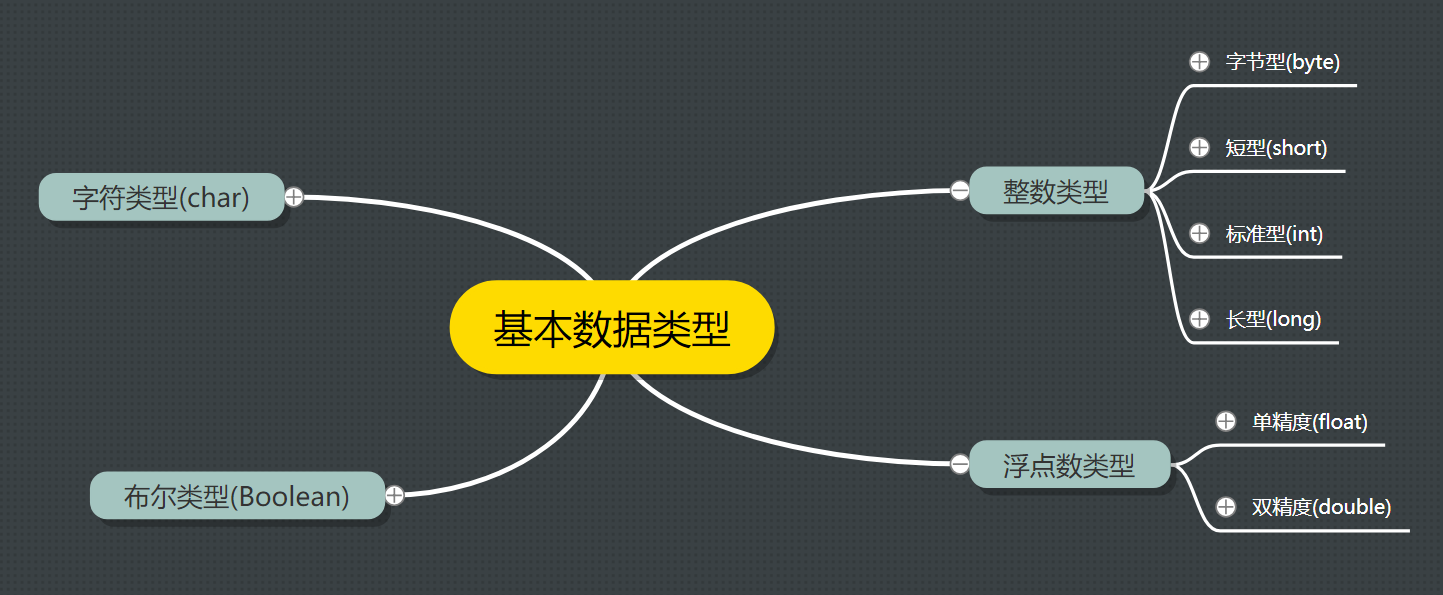9223372036854775807

1.79769313486231570E+308

二.各類基本資料之間的轉換

1.自動轉換(它只能按優先關係將位數少的資料型別向位數多的資料型別轉換)

Byte、short、char → int → long → float → double

Byte、int int int
Byte、short、int long long
Byte、short、int、long float float
Byte、short、int、long、float double double
char int int

public class practice {

public static void main(String[] args) {
char c = 'h';
byte b = 6;
int i = 100;
long l = 567L;
float f = 8.99f;// 單精度小數需用F或f為字尾
double d = 4.7788;// 雙精度小數可用D、d或不加字尾表示

int aa = c + i;// c自動轉換為int型別再運算
long ll = l - aa;// aa自動轉換為long型別再運算
float ff = b * f;// b自動轉換為float型別再運算
double dd = ff / aa + d;// aa自動轉換為float型別運算，ff/aa得float結果後再自動轉換為double型別再進行運算

System.out.println("aa=" + aa);
System.out.println("ll=" + ll);
System.out.println("ff=" + ff);
System.out.println("dd=" + dd);
}

}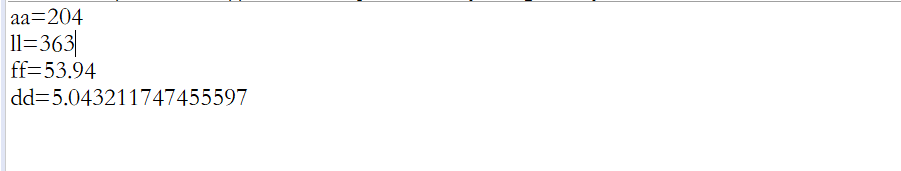2.強制轉換：將高階資料轉換成低階資料(其中的char型別與int型別轉換有些特殊)

public class practice {

public static void main(String[] args) {
char c = 'h';
byte b = 6;
int i = 100;
long l = 567L;
float f = 8.99f;// 單精度小數需用F或f為字尾
double d = 4.7788;// 雙精度小數可用D、d或不加字尾表示

int ii=(int)l;//將long型的l強制轉換為int型的ii
long ll=(long)f;//將float型的f強制轉換為long型的ll
int cc=(int)c;//將char型的c強制轉換為int型的cc

System.out.println("ii=" + ii);
System.out.println("ll=" + ll);
System.out.println("cc=" + cc);

}

}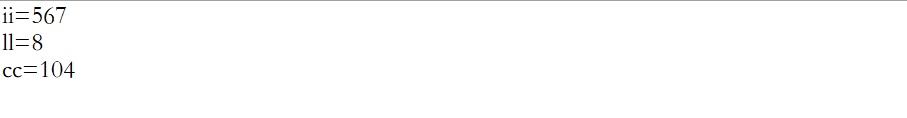• 【問題來了】：當char型數字想要轉換為int型或int型轉換為char型還用同樣方法可以嗎？答案是：NO！

​ 當int型數字轉換為char型則得不出結果

public class practice {

public static void main(String[] args) {
char c = '8';
int i = 9;
int b1 = c;//將char型數字自動轉換為int型
char cc1 = (char) i;//將int型數字強行轉換為char型
System.out.println("b1=" + b1);//56，即8的ASCII碼
System.out.println("cc1=" + cc1);//沒有結果
}

}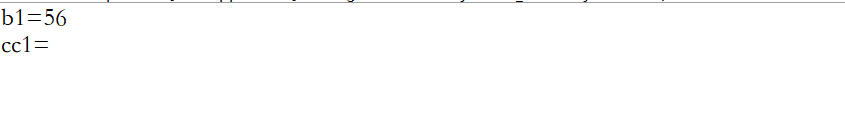• 由char型數字轉int型，給字元減'0'即可

• 由int型轉char型，給數字加'0'並強制轉換即可

public class practice {

public static void main(String[] args) {
char c = '8';
int i = 9;

int b = c - '0';//由char型轉int型，給字元減'0'即可
char cc = (char) (i + '0');//由int型轉char型，給數字加'0'並強制轉換即可
System.out.println("b=" + b);
System.out.println("cc=" + cc);
}

}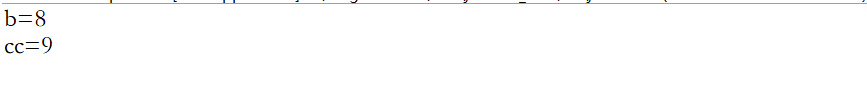三.基本資料型別和String之間轉換(附：物件包裝類及所對應的基本資料型別)

Boolean boolean
Byte byte
Character char
Short short
Integer int
Long long
Float float
Double double

1.字串→基本資料型別(以字串→int型，float型為例)

• 呼叫包裝類的 對應的包裝類.parsexxx 方法
• 呼叫包裝類的 對應的包裝類.valueOf() 方法

public class practice {

public static void main(String[] args) {
String str = "12345678";
int a = Integer.parseInt(str);//第一種方法
int a1 = Integer.valueOf(str);//第二種方法
float f = Float.parseFloat(str);//第一種方法
float f1=Float.valueOf(str);//第二種方法
System.out.println(a);//12345678
System.out.println(a1);//12345678
System.out.println(f);//1.2345678E7
System.out.println(f1);//1.2345678E7
}
}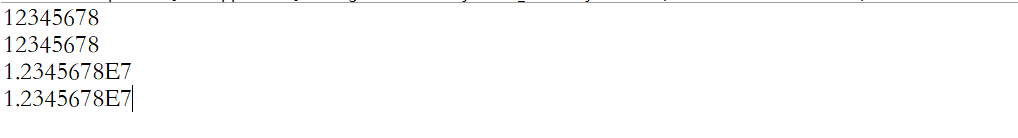2.基本資料型別→字串(以int型→字串為例)

• 用一個空字串加上基本型別，得到的就是基本型別資料對應的字串
• 使用String類的 String.valueOf() 方法
• 使用包裝類的 對應的包裝類.toString() 方法

public class practice {

public static void main(String[] args) {
int a = 125;
String str = " ";//方法一
String str1 = a + str;//方法二
String str2 = String.valueOf(a);//方法三
String str3 = Integer.toString(a);
System.out.println(str1);//125
System.out.println(str2);//125
System.out.println(str3);//125

}
}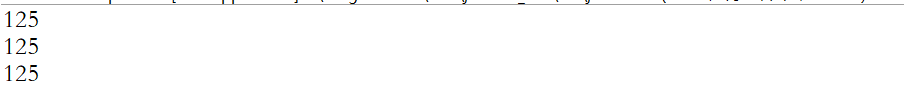四.String與字元陣列轉換

1.字元陣列→字串

• public static String valueOf(char[]) 用字元陣列中的全部字元建立字串物件。
• public static valueOf String(char[]，int offset，int count) 用字元陣列中的全部字元和部分字元建立字串物件。(注：data - 字元陣列。offset - String 的索引【字串索引從0開始】。count - String 值的長度。)
• String(char[]) 用字元陣列中的全部字元建立字串物件。
• String(char[]，int offset，intlength) 用字元陣列中的部分字元建立字串物件。

public class practice {

public static void main(String[] args) {
char[] arr = { 'h', 'e', 'l', 'l', 'o' };
String str = String.valueOf(arr);//第一種方法
String str1=String.valueOf(arr, 0, 3);//第二種方法
String str2 =new String(arr);//第三種方法
String str3=new String(arr, 0, 3);//第四種方法
System.out.println(str);//hello
System.out.println(str1);//hel(其中不包含下標為3的字元)
System.out.println(str2);//hello
System.out.println(str3);//hel(其中不包含下標為3的字元)
}
}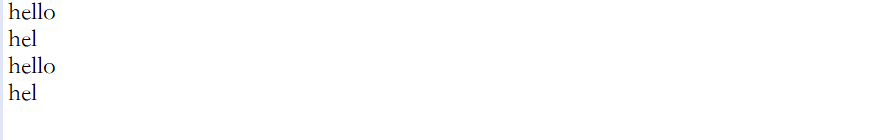2.字串→字元陣列

• public char[] toCharArray()：將字串中的全部字元存放在一個字元陣列中的方法。
• public void getChars(int srcBegin, int srcEnd, char[] dst, int dstBegin)：提供了將指定索引範圍內的字串存放到陣列中的方法

package Sort;

import java.util.Arrays;

public class practice {

public static void main(String[] args) {
char[ ] arr = new char;
char[ ] arr1 = new char;
String str = "hello";
arr=str.toCharArray();
str.getChars(0, 3, arr1, 0);//要複製的第一個字元位於索引 srcBegin 處； 要複製的最後一個字元位於索引 srcEnd-1 處（因此要複製的字元總數是srcEnd-srcBegin）。 要複製到 dst 子陣列的字元從索引 dstBegin 處開始，並結束於索引： dstbegin + (srcEnd-srcBegin) - 1
System.out.println(Arrays.toString(arr));//[h, e, l, l, o]
System.out.println(Arrays.toString(arr1));//[h, e, l]
}
}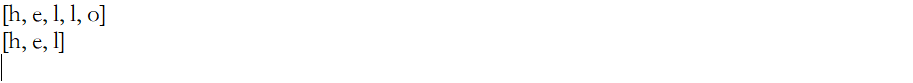五.String與位元組陣列轉換

1.位元組陣列→字串

• String(byte[])：通過使用平臺的預設字符集解碼指定的 byte 陣列，構造一個新的 String。
• String(byte[]，int offset，int length) ：用指定的位元組陣列的一部分，即從陣列起始位置offset開始取length個位元組構造一個字串物件。

package Sort;

import java.util.Arrays;

public class practice {

public static void main(String[] args) {
byte[] arr = { 'h', 'e', 'l', 'l', 'o' };
String str1=new String(arr);//第一種方法
String str2=new String(arr, 0, 3);//第二種方法
System.out.println(str1);//hello
System.out.println(str2);//hel(其中不包含下標為3的字元)
}
}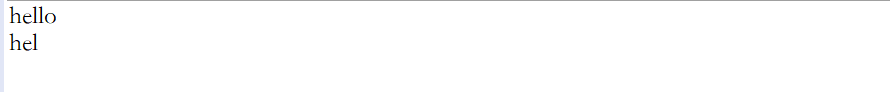2.字串→位元組陣列

• public byte[] getBytes() ：使用平臺的預設字符集將此 String 編碼為byte 序列，並將結果儲存到一個新的 byte 陣列中。
• public byte[] getBytes(String charsetName) ：使用指定的字符集將此 String 編碼到 byte 序列，並將結果儲存到新的 byte 陣列。注：charsetName為編碼字符集

package Sort;

import java.io.UnsupportedEncodingException;
import java.util.Arrays;

public class practice {

public static void main(String[] args) throws UnsupportedEncodingException {
byte[] arr = new byte;
byte[] arr1 = new byte;
String str = "hello";
arr = str.getBytes();//第一種方法
arr1 = str.getBytes("UTF-8");//第二種方法
System.out.println(Arrays.toString(arr));// [104, 101, 108, 108, 111]
System.out.println(Arrays.toString(arr1));// [104, 101, 108, 108, 111]
}
}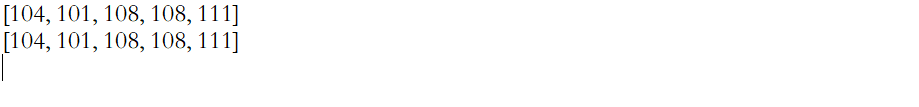[Image_Link]https://img-blog.csdnimg.cn/20200217230246440.jpg?x-oss-process=image/watermark,type_ZmFuZ3poZW5naGVpdGk,shadow_10,text_aHR0cHM6Ly9ibG9nLmNzZG4ubmV0L1FSUlFRUg==,size_16,color_FFFFFF,t_7